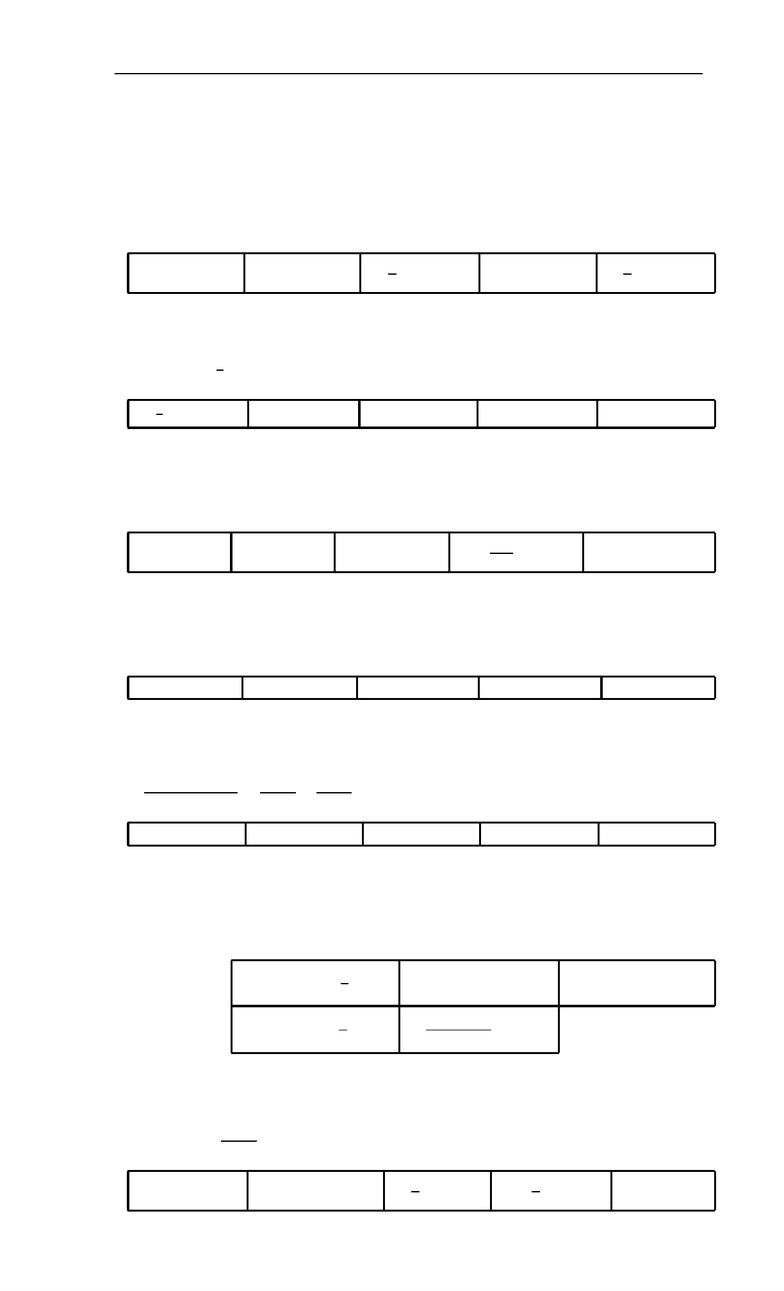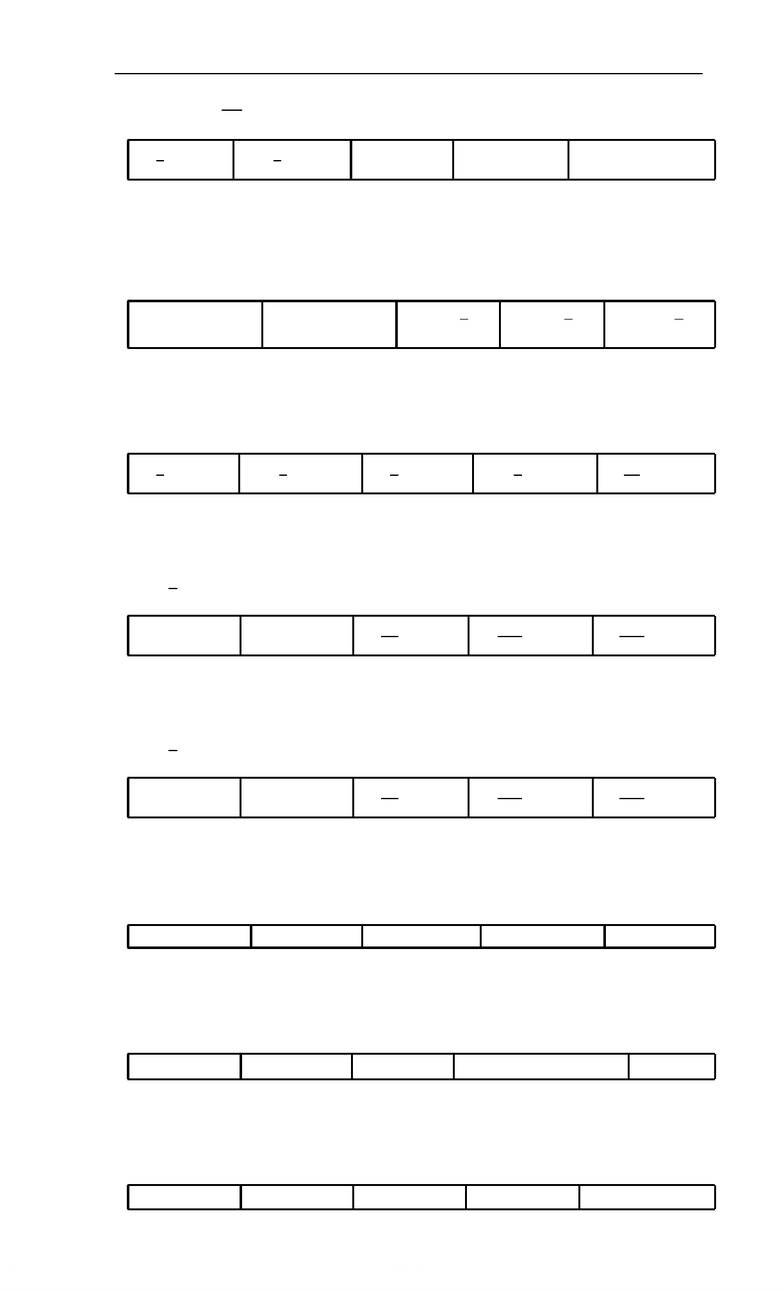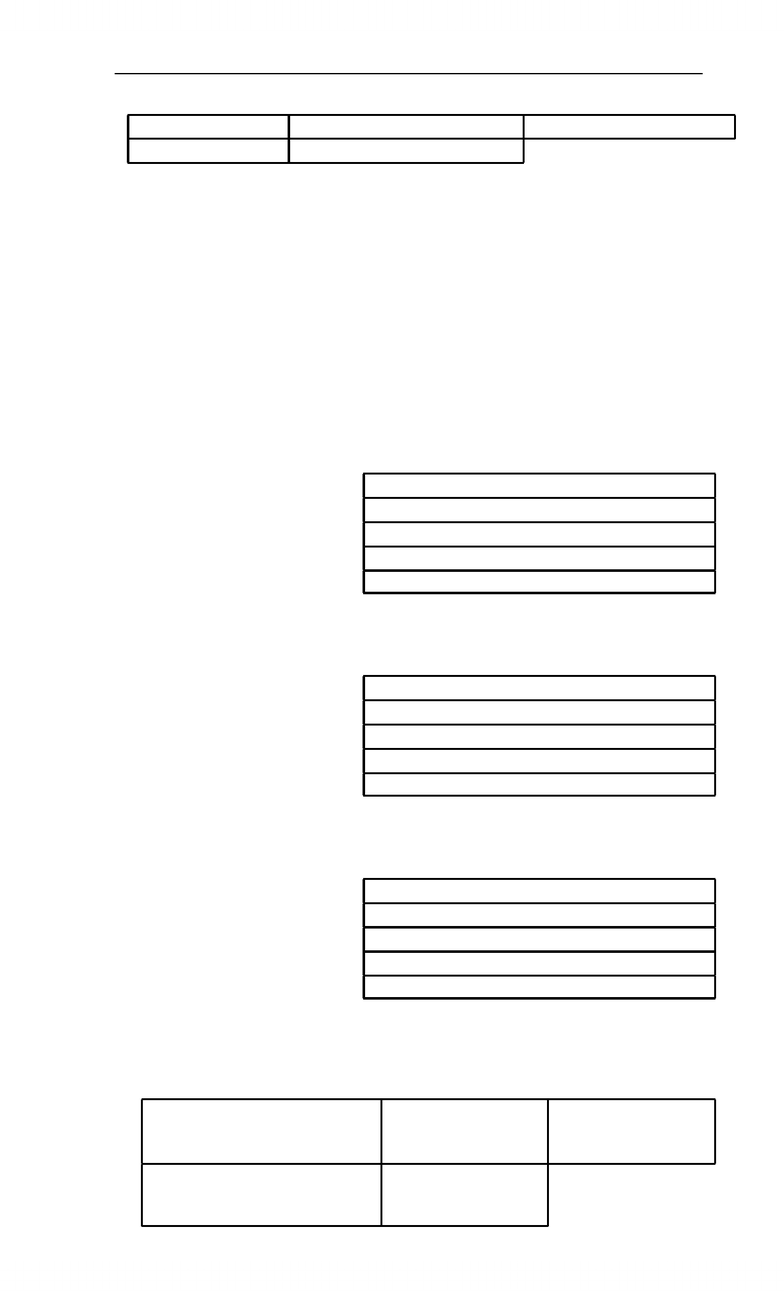# Mathematics 1225A/B Study Guide - Final Guide: Saddle Point, Maxima And Minima, Lagrange Multiplier

51 views9 pagesfrom 2011 tests
Page 1 CODE 111 Mathematics 1225B
Practice Final Exam
PART A (35 marks)
IN THIS BOOKLET. Only the (scantron) answer sheet will be marked for Part
A. Extra time will NOT be given for coding answers at the end of the exam.
A1. If log3xlog3x2= 2, ﬁnd x.
A: 6 B: 3 C:1
3D: 9 E:1
9
A2. If f(x) = 4ex, ﬁnd f(4).
A:1
2e2B:e2C: 2e2D: 4e2E: 8e2
A3. If f(x) = 2x, ﬁnd f(0).
A: 0 B: 1 C:1D:
1
ln 2 E:ln 2
A4. Find the slope of the tangent line to the graph of y=e2xat the point where x= ln 3.
A: 8 B: 6 C: 12 D: 18 E: 9
A5. If 8x11
(x2)(x1) =A
x2+B
x1, ﬁnd B.
A: 1 B: 2 C: 3 D: 4 E: 5
A6. Find Z12x3ln x dx.
A:x43 ln x
1
4+CB: 3x3(xln x1) + CC: 12x4(ln x1) + C
D: 3x4ln x
1
4+CE:3x4(ln x)2
2+C
A7. Evaluate Ze3
e
1
xln xdx.
A: ln 3 B:e3eC:1
3D:
2
3E: 0
Unlock document

This preview shows pages 1-3 of the document.
Unlock all 9 pages and 3 million more documents.Mathematics 1225B
Practice Final Exam CODE 111 from 2011 tests
Page 2
A8. Evaluate Z
1
1
x5dx.
A:1
4B:
1
4C: 1 D:1E: diverges
A9. Determine which one of the following integrals represents the area of the region bounded
by y=x2and y= 4.
A:Z2
0
(4 x2)dx B:Z4
0
(4 x2)dx C:Z2
0
y dy D:Z4
0
y dy E:Z4
0
2y dy
A10. Find the area of the region bounded by y=x2xand y=x.
A:8
3B:
8
3C:4
3D:
4
3E:16
3
A11. Find the volume of the solid of revolution obtained by rotating the region bounded by
y=x,y= 2 and x= 0 about the x-axis.
A: 6πB: 8πC:8π
3D:16π
3E:32π
5
A12. Find the volume of the solid of revolution obtained by rotating the region bounded by
y=x,y= 2 and x= 0 about the y-axis.
A: 6πB: 8πC:8π
3D:16π
3E:32π
5
A13. If f(x, y) = x23xy +y3, ﬁnd fy(3,1).
A:7B: 3 C: 24 D:6E: 1
A14. If f(x, y) = yx, ﬁnd fyx(x, y).
A:yxln yB:xyx1C:yx1D:yx1(1 + xln y)E:yx
A15. Let f(x, y) = yex3xy. Find the only critical point of f(x, y).
A: (0,0) B: (0,3) C: (1,1) D: (3,1) E: (3,1)
Unlock document

This preview shows pages 1-3 of the document.
Unlock all 9 pages and 3 million more documents.from 2011 tests
Page 3 CODE 111 Mathematics 1225B
Practice Final Exam
A16. Find all the critical points of the function f(x, y) = 3xx33xy2.
A: (0,0) B: (1,1),(1,1) C: (1,0),(1,0),(0,1),(0,1)
D: (0,0),(1,1),(1,1) E: (1,1),(1,1),(1,1),(1,1)
Use the following information for questions 17, 18 and 19.
f(x, y) = x36xy y2
fx(x, y) = 3x26y
fy(x, y) = 6x2y
fxx(x, y) = 6x
fyy(x, y) = 2
fxy(x, y) = 6
A17. Which one of the following is true for the point (1,3)?
A: (1,3) is not a critical point of f(x, y).
B:f(x, y) has a saddle point at (1,3).
C:f(x, y) has a local minimum at (1,3).
D:f(x, y) has a local maximum at (1,3).
E: The second partials test yields no information.
A18. Which one of the following is true for the point (6,18)?
A: (6,18) is not a critical point of f(x, y).
B:f(x, y) has a saddle point at (6,18).
C:f(x, y) has a local minimum at (6,18).
D:f(x, y) has a local maximum at (6,18).
E: The second partials test yields no information.
A19. Which one of the following is true for the point (0,0)?
A: (0,0) is not a critical point of f(x, y).
B:f(x, y) has a saddle point at (0,0).
C:f(x, y) has a local minimum at (0,0).
D:f(x, y) has a local maximum at (0,0).
E: The second partials test yields no information.
A20. If the method of Lagrange multipliers is used to maximize the function f(x, y) = xy + 10
subject to the constraint x2+ 9y2= 18, what system of equations must be solved?
A:xy + 10 + λ(x2+ 9y218) = 0 B: 2x+λy = 0
18y+λx = 0
xy + 10 = 0
C:y+ 2= 0
x+ 18yλ = 0
x2+ 9y218 = 0
D:xy + 2λx = 0
xy + 2λy = 0
x2+ 9y218 = 0
E:xy + 10 = 0
x2+ 9y218 = 0
Unlock document

This preview shows pages 1-3 of the document.
Unlock all 9 pages and 3 million more documents.